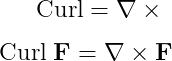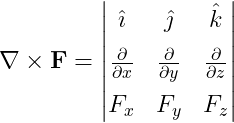# How to write a curl operator(∇×F) in LaTeX?

Curl operator is like a divergence operator. However, in the case of curl, there will be a cross product between gradient and vector instead of the dot product.

\documentclass{article}
\begin{document}
$\textup{Curl}=\nabla\times$
$\textup{Curl}\;\textbf{F}=\nabla\times\textbf{F}$
\end{document}

Output :In latex, the best practice is to use the physics package for curl symbol as well, because the physics package contains a pre-defined \curl command that denotes the entire curl operator.

\documentclass{article}
\usepackage{physics}
\begin{document}
$\curl$
$\curl{\vb{F}}$
$\curl(\vb{F_{1}}+\vb{F_{2}})$
$\qty(\pdv{x}\hat{\imath}+\pdv{y}\hat{\jmath}+\pdv{z}\hat{k})\cp \vb{F}$
\end{document}

Output :And Curl operation is also written as a matrix from. For this, you need to take the help of matrix environment. And for matrix, you can use both amsmath or physics packages.

\documentclass{article}
\usepackage{amsmath,physics}
\begin{document}
$\nabla \cp \vb{F}= \begin{vmatrix} \vu{\imath} & \vu{\jmath} & \hat{k}\\ \pdv{x} & \pdv{y} & \pdv{z}\\ F_{x} & F_{y} & F_{z} \end{vmatrix}$
\end{document}

Output :#### Md Jidan Mondal

LaTeX expert with over 10 years of experience in document preparation and typesetting. Specializes in creating professional documents, reports, and presentations using LaTeX.